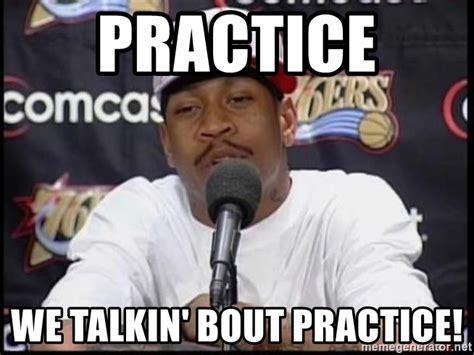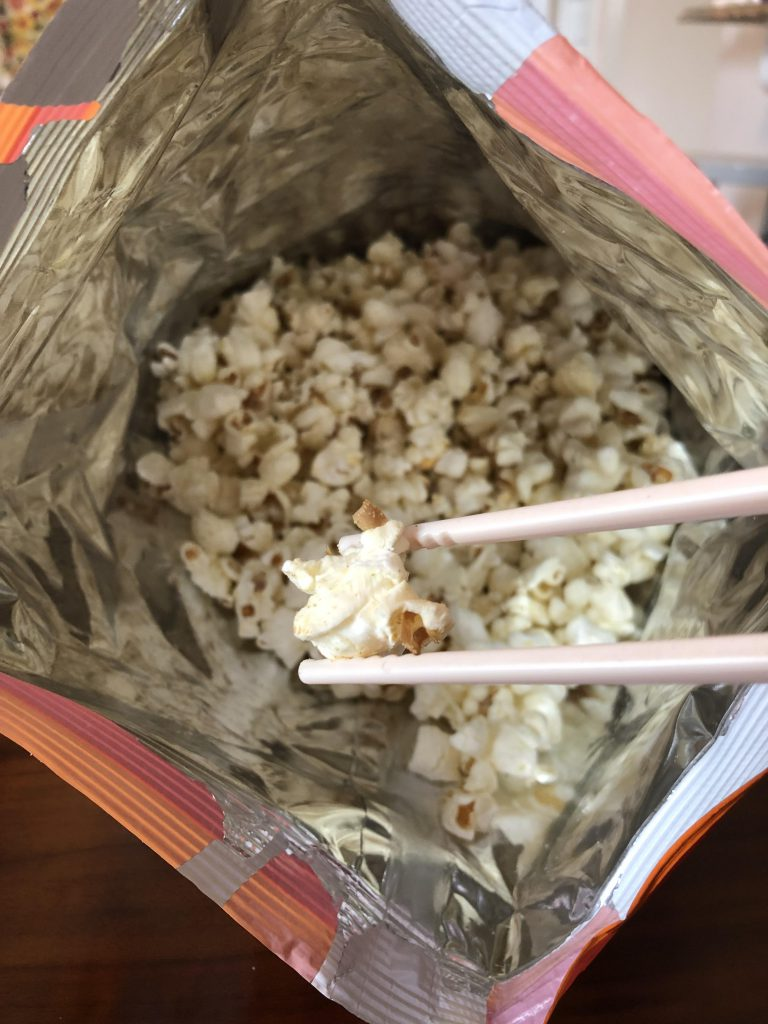# Hand Calculation## Practice: One-Way ANOVA

In this activity, students will take data from a fictitious multi-group design to practice conducting a One-way Analysis of Variance (ANOVA). First, provide students with the research scenario and the accompanying questions to have them determine the research design, statistical analysis to use, and independent and dependent variables. Next, have students set up a data …## Practice: t-test for Independent Means

In this activity, students will take data from a fictitious two-group design to practice conducting a t-test for Independent Means. First, provide students with the research scenario and the accompanying questions to have them determine the research design, statistical analysis to use, and independent and dependent variables. Next, have students set up a data file …## Lab Activity: How Does Novelty Impact Food Enjoyment? (t-test for Independent Means/Samples)

In this activity (created by Dr. Eliane Boucher), students will participate in a simplified version of the first study conducted by O’Brien and Smith (2019). In this study, participants were instructed to eat a familiar food (popcorn) in a conventional or an unconventional way (with chopsticks). Participants then rated how enjoyable the food was (note …

## Practice: Calculating the Pearson r Correlation (Video Games and GPA)

Instructors should assign this problem (about how many hours per week they played video games and their GPA) to students as in-class practice or homework after students have learned how to calculate a Pearson r correlation and test for significance. The activity leads students through determining the hypotheses, calculating the correlation coefficient, making a decision …

## Practice: Calculating the Pearson r Correlation (Math and Spelling Ability)

Instructors should assign this problem (about scores on a math and a spelling test) to students as in-class practice or homework after students have learned how to calculate a Pearson r correlation and test for significance. The activity leads students through determining the hypotheses, calculating the correlation coefficient, making a decision about the null hypotheses …

## Practice: Calculating the Pearson r Correlation (Height and Running Speed)

Instructors should assign this problem (about how height and running speed in the 40 yard dash) to students as in-class practice or homework after students have learned how to calculate a Pearson r correlation and test for significance. The activity leads students through determining the hypotheses, calculating the correlation coefficient, making a decision about the …

## Practice: Calculating the t-test for Independent Samples/Means (Temperature and Mood)

Instructors should assign this problem (about how temperature impacts mood) to students as in-class practice or homework after students have learned how to calculate a t-test for independent means and test for significance. The activity leads students through determining the type of statistical analysis to use, the hypotheses, calculating the t-ratio and effect size (if …

## Practice: Calculating the t-test for Independent Samples/Means (Research Methods and GPA)

Instructors should assign this problem (about how taking research methods impacts GPA) to students as in-class practice or homework after students have learned how to calculate a t-test for independent means and test for significance. The activity leads students through determining the type of statistical analysis to use, the hypotheses, calculating the t-ratio and effect …

## Practice: Calculating the t-test for Paired-Samples/Dependent Means (Drugs and Learning)

Instructors should assign this problem (about drugs and learning) to students as in-class practice or homework after students have learned how to calculate a t-test for dependent means and test for significance. The activity leads students through determining the type of statistical analysis to use, the hypotheses, calculating the t-ratio and effect size (if appropriate), …

## Lecture Slides: Calculating the t-test for Independent Means/Samples (Does Eating Spinach Make Your Stronger?)

The goal of this PowerPoint is to work through the step-by-step process of hand calculating a t-test for independent means. The slides start with an explanation of the formula involved and then a sample problem is presented. It is designed for you to have the class solve the problem along with you as you advance …

## Lecture Slides: Calculating the Pearson r Correlation (Study Hours and GPA)

The goal of this PowerPoint is to work through the step-by-step process of hand calculating a Person Correlation. The slides start with the formula involved and then a sample problem is presented. It is designed for you to have the class solve the problem along with you as you advance through the slides and animation. …

## Lecture Slides: Calculating the t-test for Paired-Samples/Dependent Means (Weight Loss Study)

The goal of this PowerPoint is to work through the step-by-step process of hand calculating a t-test for dependent means. The slides start with an explanation of the formula involved and then a sample problem is presented. It is designed for you to have the class solve the problem along with you as you advance …

## Practice: Calculating the t-test for Paired-Samples/Dependent Means (Thinking Logically Over Time)

Instructors should assign this problem (about graduate programs training students to think logically) to students as in-class practice or homework after students have learned how to calculate a t-test for dependent means and test for significance. The activity leads students through determining the type of statistical analysis to use, the hypotheses, calculating the t-ratio and …